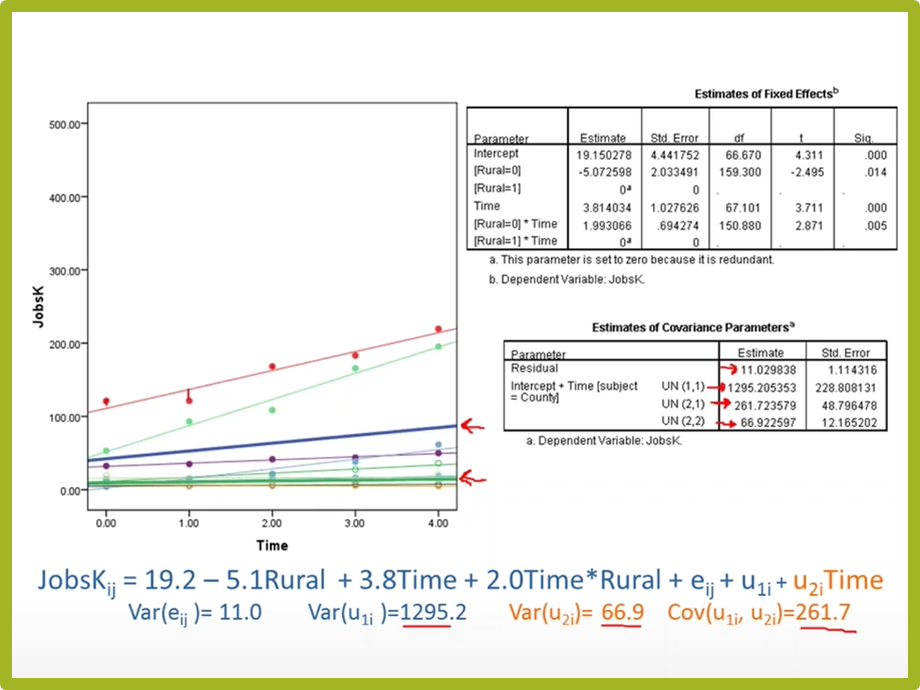Poisson and Negative Binomial Regression Models for Count Data
Learn exactly when you need to use Poisson or Negative Binomial Regression in your analysis, how to interpret the results, and how they differ from similar models.
Instructor: Jeff MeyerEver discover that your data are not normally distributed, no matter what transformation you try? It may be that they follow another distribution altogether.

Although they are numerical, discrete count data often follow a Poisson or Negative Binomial distribution, not a normal one.

Examples of discrete counts include:

- Number of tagged fish that return to a reef each month
- Number of months of unemployment
- Number of arrests in a neighborhood

This recording will give you an overview of Poisson and Negative Binomial regression models.

Covered in this webinar:

1.
How these regression models differ from Ordinary Linear Regression
2.
The type of data for which each is appropriate
3.
How to interpret the output from each
4.
And more!